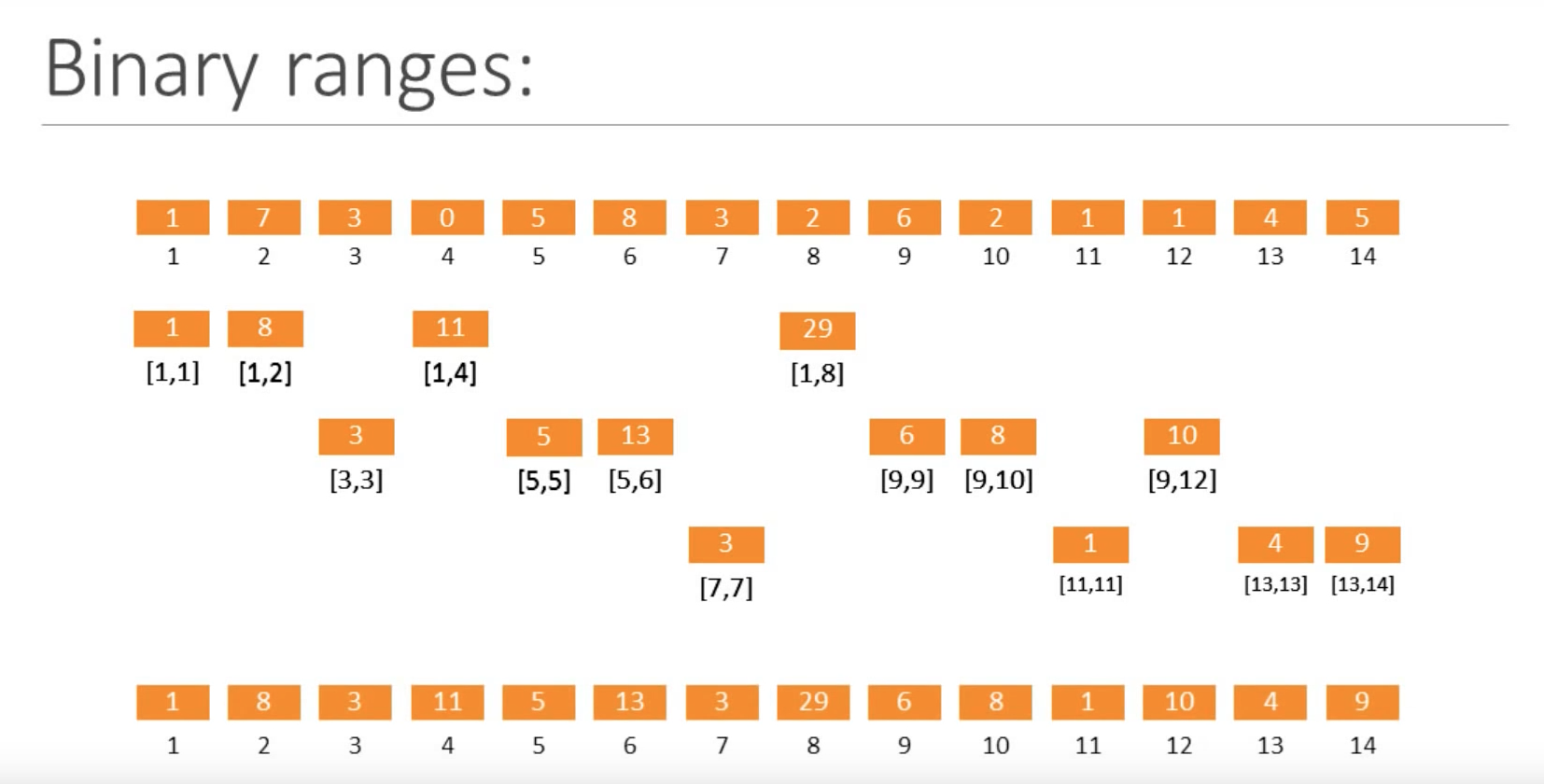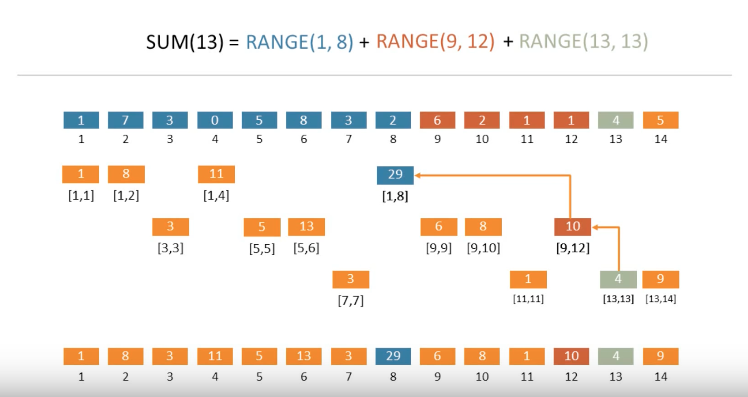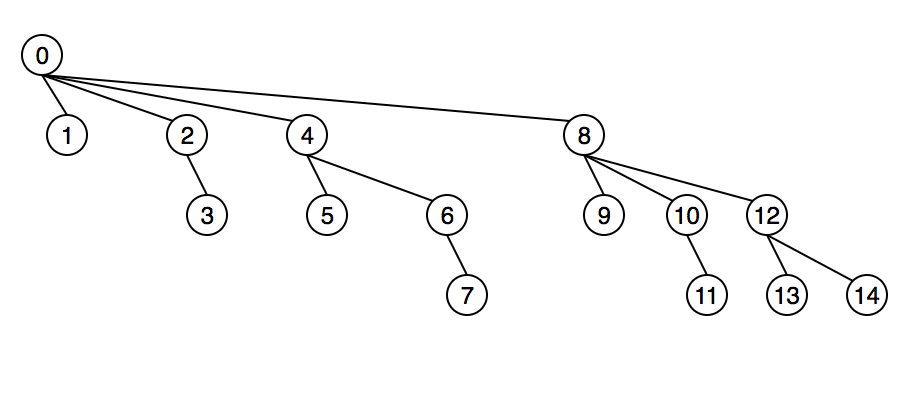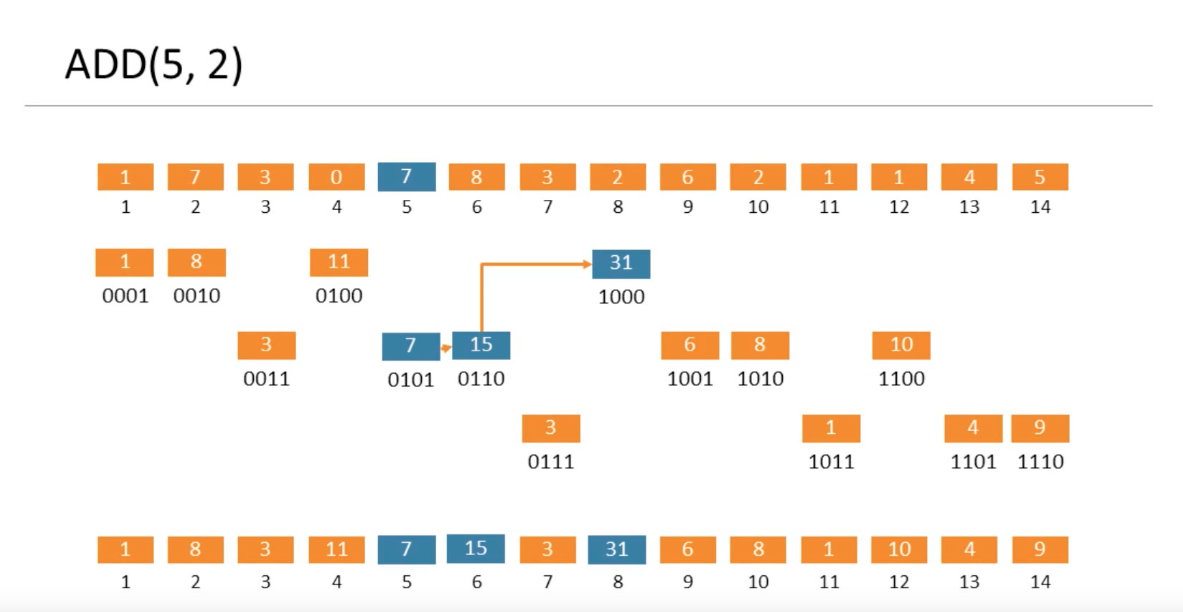# 定义

A Fenwick tree or binary indexed tree is a data structure that can efficiently update elements and calculate prefix sums in a table of numbers.

1. update(idx, delta)：将num加到位置idx的数字上。
2. prefixSum(idx)：求从数组第一个位置到第idx（含idx）个位置所有数字的和。
3. rangeSum(from_idx, to_idx)：求从数组第from_idx个位置到第to_idx个位置的所有数字的和

1. update(idx, delta)：更新操作需要更新cumulative sum数组中每一个受此更新影响的前缀和，即从idx其到最后一个位置的前缀和。该操作为O(n)时间复杂度。
2. prefixSum(idx)：直接返回cumulativeSum[idx + 1]即可。该操作为O(1)时间复杂度。
3. rangeSum(from_idx, to_idx)：直接返回cumulativeSum[to_idx + 1] - cumulativeSum[from_idx]即可。该操作为O(1)操作。

# 填坑法构造Binary Indexed Tree

Binary Indexed Tree求和的基本思想在于，给定需要求和的位置i，例如13，我们可以利用其二进制表示法来进行分段（或者说分层）求和：13 = 2^3 + 2^2 + 2^0，则prefixSum(13) = RANGE(1, 8) + RANGE(9, 12) + RANGE(13, 13) （注意此处的RANGE(x, y)表示数组中第x个位置到第y个位置的所有数字求和）。如下面例子中所示：

arr = [1, 7, 3, 0, 5, 8, 3, 2, 6, 2, 1, 1, 4, 5]
prefixSum(13) = RANGE(1, 8) + RANGE(9, 12) + RANGE(13, 13)
= 29 + 10 + 4 = 43prefixSum(13) = prefixSum(0b00001101)
= BIT + BIT + BIT
= BIT[0b00001101] + BIT[0b00001100] + BIT[0b00001000]# 利用Binary Indexed Tree求prefix sum或range sumprefixSum(13) = prefixSum(0b00001101)
= BIT + BIT + BIT
= BIT[0b00001101] + BIT[0b00001100] + BIT[0b00001000]


x = 13 = 0b00001101
-x = -13 = 0b11110011
x & (-x) = 0b00000001
x - (x & (-x)) = 0b00001100


# 更新数组中的元素

update(5, 2)为例，我们想要给原数组中第5个位置的数字加2，基于之前构造好的Binary Indexed Tree，更新的过程如下图中所示：x = 5 = 0b00000101
-x = -5 = 0b11111011
x & (-x) = 0b00000001
x + (x & (-x)) = 0b00000110


# Binary Indexed Tree的建立

Binary Indexed Tree的建立非常简单。我们只需初始化一个全为0的数组，并对原数组中的每一个位置对应的数字调用一次update(i, delta)操作即可。这是一个O(nlogn)的建立过程。

1. 初始化长度为n + 1的Binary Indexed Tree数组bit，并将list中的数字对应地放在bitbit[n]的各个位置。
2. 对于1n的每一个i，进行如下操作：
• j = i + (i & -i)，若j < n + 1，则bit[j] = bit[j] + bit[i]

# 代码实现

public class BinaryIndexedTree {
private int[] bitArr;

// O(nlogn) initialization
//	public BinaryIndexedTree(int[] list) {
//		this.bitArr = new int[list.length + 1];
//		for (int i = 0; i < list.length; i++) {
//			this.update(i, list[i]);
//		}
//	}

public BinaryIndexedTree(int[] list) {
// O(n) initialization
this.bitArr = new int[list.length + 1];
for (int i = 0; i < list.length; i++) {
this.bitArr[i + 1] = list[i];
}

for (int i = 1; i < this.bitArr.length; i++) {
int j = i + (i & -i);
if (j < this.bitArr.length) {
this.bitArr[j] += this.bitArr[i];
}
}
}

/**
* Add delta to elements in idx of original array
* @param idx index of the element in original array that is going to be updated
* @param delta number that will be added to the original element.
*/
public void update(int idx, int delta) {
idx += 1;
while (idx < this.bitArr.length) {
this.bitArr[idx] += delta;
idx = idx + (idx & -idx);
}
}

/**
* Get the sum of elements in the original array up to index idx
* @param idx index of the last element that should be summed.
* @return sum of elements from index 0 to idx.
*/
public int prefixSum(int idx) {
idx += 1;
int result = 0;
while (idx > 0) {
result += this.bitArr[idx];
idx = idx - (idx & -idx);
}

return result;
}

/**
* Get the range sum of elements from original array from index from_idx to to_idx
* @param from_idx start index of element in original array
* @param to_idx end index of element in original array
* @return range sum of elements from index from_idx to to_idx
*/
public int rangeSum(int from_idx, int to_idx) {
return prefixSum(to_idx) - prefixSum(from_idx - 1);
}
}



# Reference

08-1388707-303万+
06-253万+
03-183万+
03-28216
08-061562
04-251176
05-28161
11-141199
06-062524
08-094183
01-254万+
12-152651
06-108803
10-051347
12-039229
12-14364
09-291176
09-02299